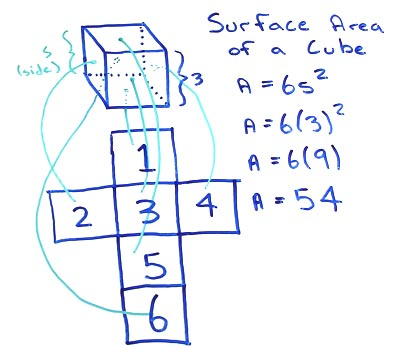# Volume of Cube

## Cube Surface Area Calculator

A cube with a side length (S) of 2 has a surface are (A) of 8.

## Cube Surface Area Formula

for cubes with a volume V and a side length S

• A = 6S2

substitute the side length S with the measured value, in this example lets use a side length of 4

• A = 6(4)2

now square the side length using the square function on your calculator or multiplying it by itself this is the area of a single square. Multiple by value by the total number of square faces, six.

• A = 96A cube is made up of six equal squares. The area of a single square is equal to the Side length squared. To determine the surface area of the whole cube multiple the surface area of one side by six (the total number of equal surfaces on the cube). A = 6S2.

### Formulas

What is the formula for the volume of a Cube?

V = S3

What is the formula for the volume of a Rectangular Prism?

V = L x W x H

### Other Formulae

for cubes with side length S

Cube Surface Area

6S2
Cube Face Diagonal
(√2)S
Cube Space Diagonal
(√3)S
S/2
All formulae...